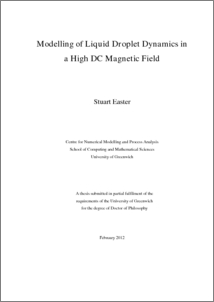# Modelling of liquid droplet dynamics in a high DC magnetic field

Easter, Stuart (2012) Modelling of liquid droplet dynamics in a high DC magnetic field. PhD thesis, University of Greenwich.Preview
PDF
Stuart_Easter_2012.pdf - Published Version

## Abstract

The oscillating droplet technique is an experimental technique that is used to measure the surface tension and viscous damping coefficients of a liquid droplet. This technique has been the subject of much analysis; theoretical, numerical, and experimental with a number of different external forces used to confine the droplet. These external forces are found to modify the oscillation frequency and damping rates, which need to be quantified in order for the measurement technique to be used. The dynamics of the droplet are three-dimensional but previous numerical work has largely focused on axisymmetric cases. This work uses numerical techniques to extend the previous analysis to include the full three-dimensional effects.

In this work a three-dimensional numerical model is designed, developed and applied to study the dynamics of a liquid droplet both in free space and with a high DC magnetic field used to balance gravitational forces. The numerical model is a grid point formulation of the pseudo-spectral collocation method discretised in a spherical coordinate system with the implicit Euler method used to advance the solution in time. A coordinate transformation method is used to ensure the direct surface tracking required for modelling the surface shape oscillations.

The study covers the laminar fluid flow regime within a droplet exhibiting translational and surface shape oscillations providing a greater understanding of the physical behaviour of the droplet along with a qualitative and quantitative comparison with theoretical results. Initially a droplet oscillating in free space is considered, with a range of surface oscillation modes used to demonstrate the three-dimensional dynamics. Then the influence of electromagnetic forces on a diamagnetic droplet is studied, this includes the field from a solenoid magnet used to levitate a diamagnetic droplet. Finally the dynamics of an electrically conducting droplet in an external static magnetic field are modelled. In each case a number of methods are used to analyse the surface displacement in order to determine the surface tension and viscous damping coefficients.

The numerical study of a freely oscillating droplet shows good agreement with the low order theoretical results for droplets in the limit of low viscosity. The high accuracy of the surface tracking method allows the non-linear effects of mode coupling and frequency shift with amplitude to be observed. There is good agreement with the theoretical values available for inviscid axisymmetric oscillations and the numerical study provides the opportunity to determine these effects for three-dimensional viscous oscillations.

The magnetic field from a solenoid is used to study the levitation of a diamagnetic droplet and the oscillation frequencies of the droplet are compared with a theoretical model. The magnetic field is analysed and the accuracy of the field calculation used when determining the modification to the oscillation frequencies is considered with the use of a theoretical model. Analysis is made into the splitting of the frequency spectrum due to the magnetic field.

The theoretical model that is available for an electrically conducting droplet in a static magnetic field predicts different fluid flow within the droplet and oscillation frequency and damping rate changes. These changes are compared qualitatively and quantitatively with the numerical model results with good agreement.

Item Type: Thesis (PhD) uk.bl.ethos.571449 numerical modelling, oscillating droplet technique, laminar fluid flow, electromagnetic forces, Q Science > QA Mathematics > QA76 Computer softwareQ Science > QC Physics School of Computing & Mathematical SciencesSchool of Computing & Mathematical Sciences > Centre for Numerical Modelling & Process AnalysisSchool of Computing & Mathematical Sciences > Department of Mathematical Sciences 11 Nov 2016 15:12 None None None None http://gala.gre.ac.uk/id/eprint/9149View Item